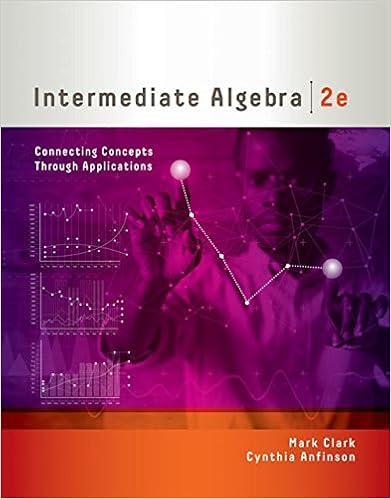# C05_Vocab Check, Highlights, Review.pdf - 326 CHAPTER 5...

• Notes
• justacult
• 9

This preview shows page 1 - 3 out of 9 pages.

##### We have textbook solutions for you!
The document you are viewing contains questions related to this textbook.The document you are viewing contains questions related to this textbook.
Chapter 6 / Exercise 40
Intermediate Algebra: Connecting Concepts through Applications
ClarkExpert Verified
326 CHAPTER 5 Exponents, Polynomials, and Polynomial Functions Solve. 101. 1 x 2 + x - 6 21 3 x 2 - 14 x - 5 2 = 0 102. 1 x 2 - 9 21 x 2 + 8 x + 16 2 = 0 With new plug-in hybrid autos and electric autos, it is very difficult to predict future sales of hybrids. One forecast is the equation y = 3 x 2 - 25 x + 345 , where x is the number of years past 2007 and y is the number of hybrid sales in thousands. Use this equation for Exercises 103 and 104. 103. Let y = 317 and solve the resulting quadratic equation by factoring. 104. Write a sentence explaining the meaning of the larger so- lution of Exercise 103. 105. Explain how solving 21x-321x-12=0 differs from solving 2x1x-321x-12=0. 1. A(n) is a finite sum of terms in which all variables are raised to nonnegative integer powers and no vari- ables appear in any denominator. 2. is the process of writing a polynomial as a product. 3. are used to write repeated factors in a more compact form. 4. The is the sum of the exponents on the variables contained in the term. 5. A(n) is a polynomial with one term. 6. If a is not 0, a 0 = . 7. A(n) is a polynomial with three terms. 8. A polynomial equation of degree 2 is also called a(n) . 9. A positive number is written in if it is written as the product of a number a , where 1 a 6 10, and a power of 10. 10. The is the largest degree of all its terms. 11. A(n) is a polynomial with two terms. 12. If a and b are real numbers and a # b = , then a = 0 or b = 0. 106. Explain why the zero factor property works for more than two numbers whose product is 0. 107. Is the following step correct? Why or why not? 108. Are the following steps correct? Why or why not? 109. 5, 3 110. 6, 7 111. - 1, 2 112. 4, - 3 113. Draw a function with intercepts 1 - 3, 0 2 , 1 5, 0 2 , and (0, 4). 114. Draw a function with intercepts 1 - 7, 0 2 , a - 1 2 , 0 b , (4, 0), and 1 0, - 1 2 .
##### We have textbook solutions for you!
The document you are viewing contains questions related to this textbook.The document you are viewing contains questions related to this textbook.
Chapter 6 / Exercise 40
Intermediate Algebra: Connecting Concepts through Applications
ClarkExpert Verified
Chapter 5 Highlights 327 DEFINITIONS AND CONCEPTS EXAMPLES Section 5.1 Exponents and Scientific Notation Product rule: a m # a n = a m + n Zero exponent: a 0 = 1, a 0 Quotient rule: a m a n = a m - n , a 0 Negative exponent: a - n = 1 a n , a 0 A positive number is written in scientific notation if it is written as the product of a number a , where 1 a 6 10, and an integer power of 10: a * 10 r . x 2 # x 3 = x 5 7 0 = 1, 1 - 10 2 0 = 1 y 10 y 4 = y 10 - 4 = y 6 3 - 2 = 1 3 2 = 1 9 , x - 5 x - 7 = x - 5 - 1 - 7 2 = x 2 Numbers written in scientific notation 568,000 = 5.68 * 10 5 0.0002117 = 2.117 * 10 - 4 Section 5.2 More Work with Exponents and Scientific Notation Power rules: ( a m ) n = a m # n ( ab ) m = a m b m a a b b n = a n b n , b 0 (7 8 ) 2 = 7 16 (2 y ) 3 = 2 3 y 3 = 8 y 3 a 5 x - 3 x 2 b - 2 = 5 - 2 x 6 x - 4 = 5 - 2 # x 6 - 1 - 4 2 = x 10 5 2 , or x 10 25 Section 5.3 Polynomials and Polynomial Functions A polynomial is a finite sum of terms in which all variables have exponents raised to nonnegative integer powers and no variables appear in a denominator.
•••